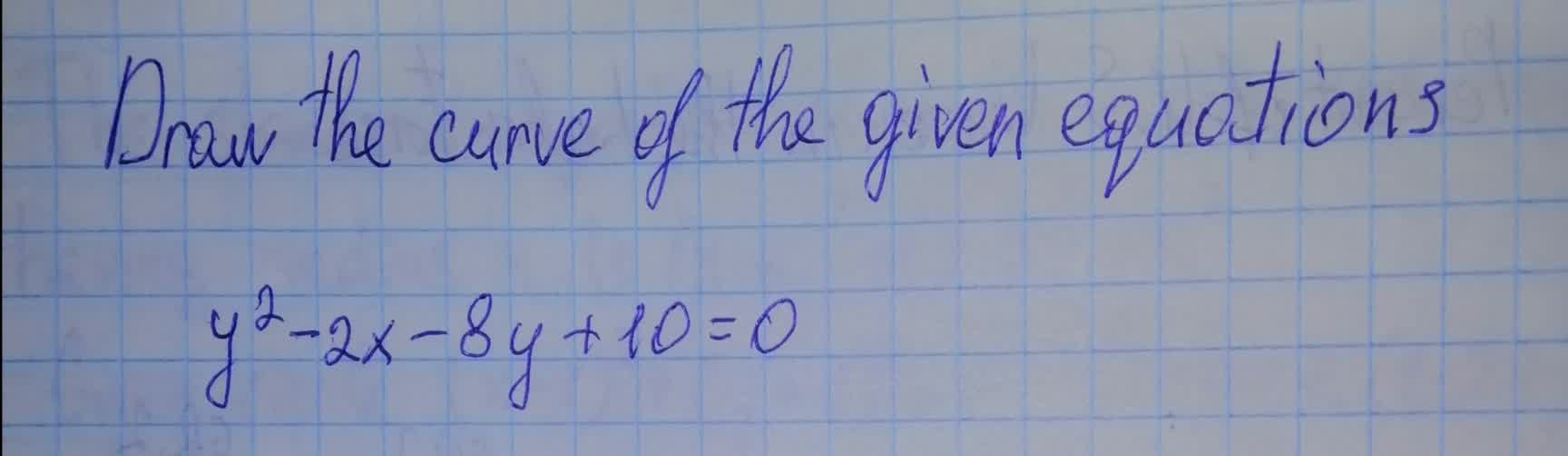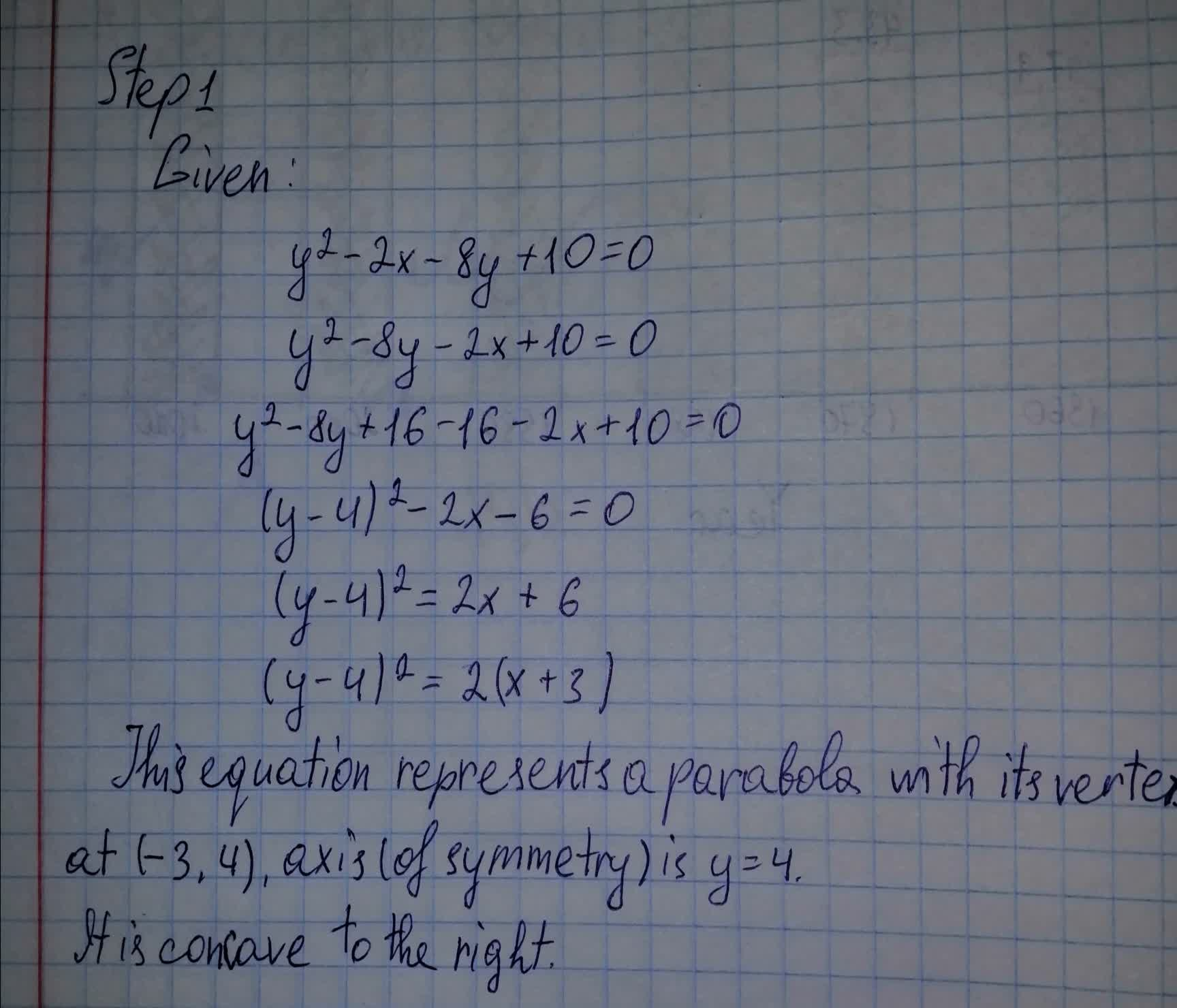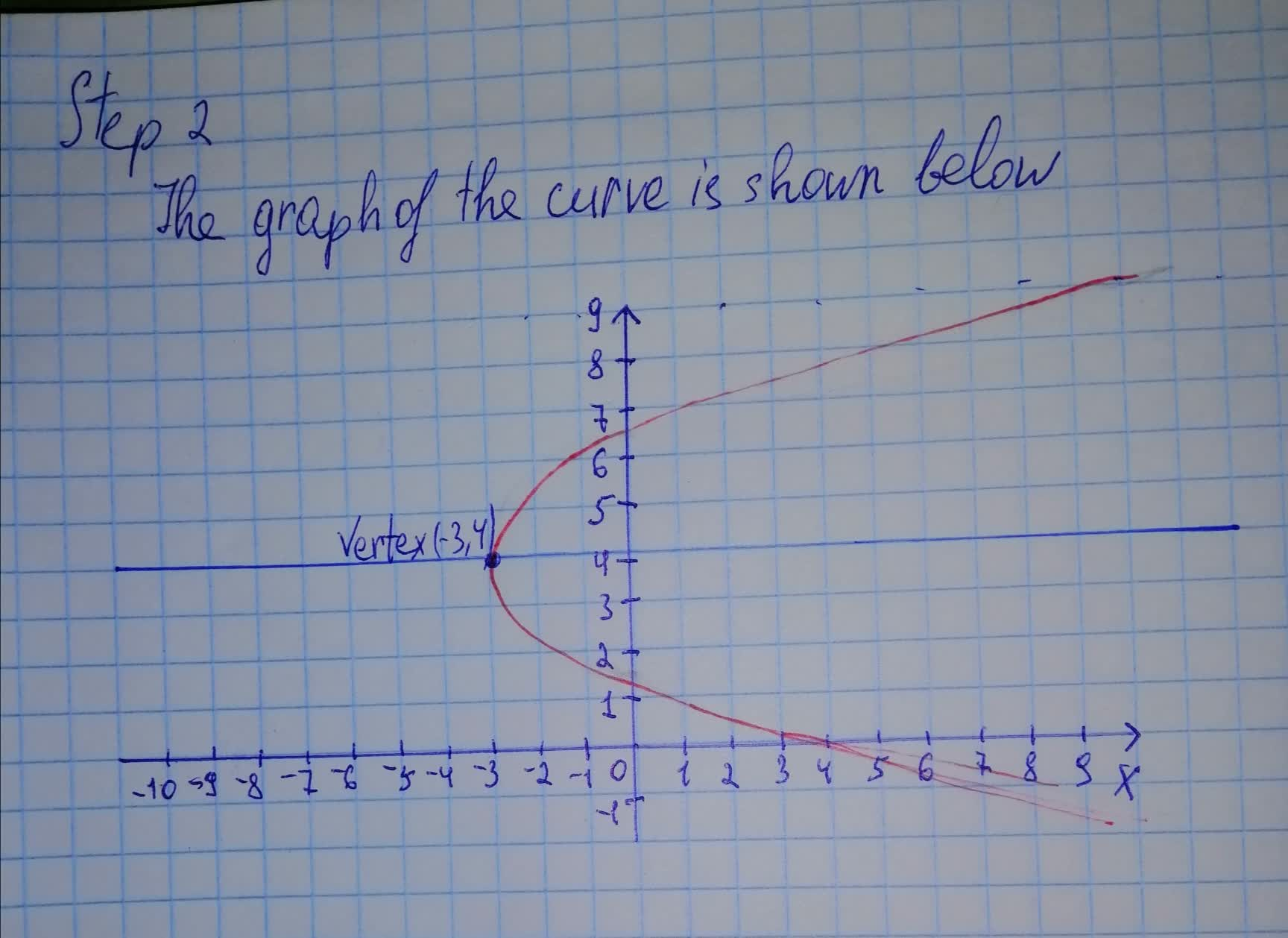Draw the curve of the given equations a. x^2+y^2+6x-12y+29=0, b. y^2-wx-8y+10=0usagirl007A 2021-08-08 Answered
Draw the curve of the given equations a. $$\displaystyle{x}^{{2}}+{y}^{{2}}+{6}{x}-{12}{y}+{29}={0}$$, b. $$\displaystyle{y}^{{2}}-{w}{x}-{8}{y}+{10}={0}$$• Questions are typically answered in as fast as 30 minutes

Solve your problem for the price of one coffee

• Math expert for every subject
• Pay only if we can solve itcomentezq

Given that: